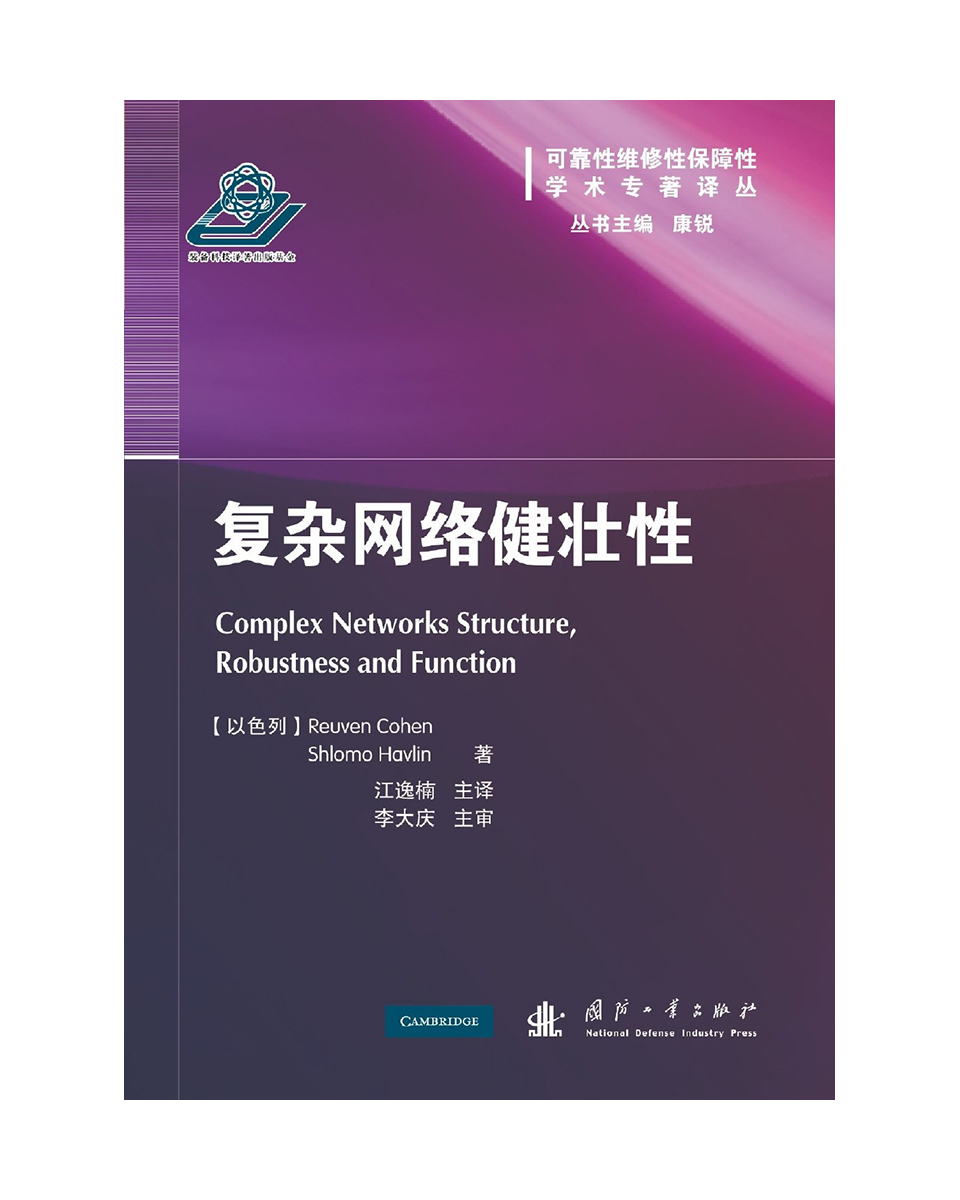Complex System Reliability Lab
English

# Complex Networks Struture, Robustness and Function

2021-01-01 02:00Name：Complex Networks Struture, Robustness and Function

ISBN9787118098488

Publisher：National Defense Industry Press

Author：Daqing Li, Yinan Jiang

Date：2015-03-01

Page：192

Introduction: This book introduces the research background, models and applications of complex networks from simple to deep, from easy to difficult. The contents are as follows: the first part is the introduction, which introduces the basic knowledge needed to study complex networks, including the brief introduction of graph theory, scale-free and fractal; the second part introduces the network model, including network model, actual network characteristics and complex network model The third part mainly introduces the structure and robustness of complex network, including the distance in scale-free network, the self similarity of complex network, the structure of percolation and directed weighted complex network, etc.; the fourth part is the function analysis of complex network, including the dynamic analysis and application of complex network propagation, immunity and search.